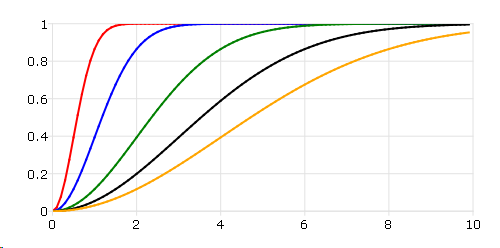# cdfRayleigh¶

## Purpose¶

Computes the Rayleigh cumulative distribution function.

## Format¶

p = cdfRayleigh(x, scale)
Parameters
• x (NxK matrix, Nx1 vector or scalar) – Values at which to evaluate the cumulative distribution function for the Rayleigh distribution. $$x \geq 0$$.

• scale (NxK matrix, Nx1 vector or scalar) – Scale parameter, ExE conformable with x. $$scale > 0$$.

Returns

p (NxK matrix, Nx1 vector or scalar) – Each element in p is the cumulative distribution function of the Rayleigh distribution evaluated at the corresponding element in x.

## Examples¶

Here is an example show the Rayleigh cumulative distribution plot with different scale parameters.

// Values
x = seqa(0, 0.1, 100);

// Scale
scale = 0.5~1~2~3~4;

// Call Rayleigh function
p = cdfRayleigh(x, scale);

plotxy(x, p);


After running above code,## Remarks¶

The Rayleigh cumulative distribution function is defined as

$1 − exp⁡(\frac{-x^2}{2\sigma^2})$